Electronic components – the Diode

Although my posts have generally been about microcontrollers, kits and related items, I have been rather lax in writing about electronics in general, and that magical world of wonder known as analogue electronics… i.e “Before Arduino” 🙂 So let’s go back to some of the basics. Starting with the diode

What is a diode? It is an electronic component that allows current to only flow in one direction. Before the advent of semiconductors, vacuum tube diodes were used. Thankfully no more…

A diode is comprised of two types of semiconductor crystal (usually made from silicon or germanium) that are highly refined then doped with an impurity. Depending on the impurity, the crystal can either be called an “N-type” or “P-type”. When you put an N-doped region next to a P-doped region, a diode or PN junction is formed. In our diodes, the P-region is called the anode, and the N-region is called the cathode. As you can imagine, these properties are useful, allowing current to flow only in one direction.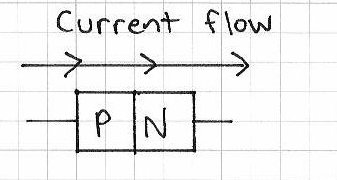The basic symbol for a diode in a circuit diagram or schematic is this: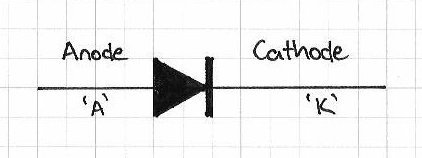So in a circuit, the current only flows in one direction, for example: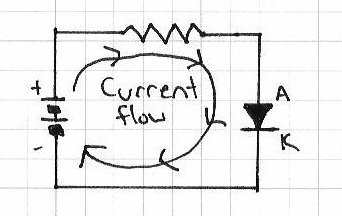When a diode is connected in this way, it is said to be forward-biased, that is the anode is connected to a higher voltage than the cathode. If the diode was reversed, with the cathode connected to the higher voltage, it would not allow current to flow, and therefore would break the circuit. A forward-biased diode is considered to be a closed switch, as the voltage does not drop as the current passes through the diode. However that is assuming the diode is perfect. And like many other things in life, it is not perfect.

All diodes are not perfect, and have what is called a forward voltage drop, this is the amount by which the voltage decreases as the current passes through the diode from anode to cathode. For silicon diodes, this is ~0.7 volts; for germanium diodes ~0.3 volts.

Diodes are also manufactured to handle a certain amount of power. Recall that:

power (watts) = current (amps) x voltage (volts)

As the voltage drop with our normal diode is 0.7V, the power dissipated by the diode can be calculated by simply multiplying the current by 0.7.

For example, if we have a 1 watt diode, how much current can it handle?

1 = current x 0.7; current = 1/0.7

Current = 1.42

So the 1 watt diode can theoretically handle 1.42 amps of current.

What happens if you use a diode the other way, that is attempt to allow current to flow from the cathode through to the anode. Ideally nothing will happen – to a point. Diodes have a breakdown voltage, when a reverse-biased (backwards) diode starts to allow current to flow through it. The breakdown voltage of each type of diode is different, it depends on the manufacturer. The best way to find out what the breakdown voltage of your diode is to check the data sheet. For example, a popular diode is the 1N4001. From page two of the data sheet (pdf), comes the following table:So for the 1N4001 diode, the breakdown voltage is 50V. Peak repetitive means that the diode can sustain doing this more than once. Excessive voltage will not usually destroy a diode. Excessive current will destroy a diode. This is interesting, as you can use a diode as a voltage regulator, provided that you don’t exceed the maximum current it can handle. Refresh your memory about voltage division with resistors. The disadvantage of using two resistors is that it can be difficult to purchase precise values.

So let’s use a zener diode instead. They are manufactured with a much more precise (and lower) voltage; and handle less power. Zener diodes have a slightly different symbol: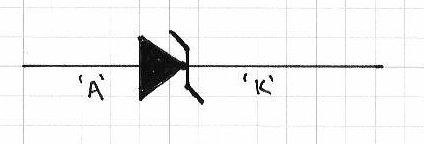Zener diodes will usually (hopefully) have their breakdown voltage within their part number. For example, an NXP 4.7V zener diode’s part number is: BZX79-B4V7. The 4V7 is the breakdown voltage, with a V for the decimal point. It can handle 500 mW, but this is not obvious – once again, you will need the data sheet (pdf). Below is a photo of a typical zener diode. It is very small, the grid paper beneath it is 5mm square. The ring or dark band around one end of the diode always indicates the cathode end:And now for an example. We have a tiny Zilog ePIR that requires a nice smooth 3.3v DC, and only draws 10mA, however the power rail on our prototype is 5V. This is a job for a 3.3V zener diode. Here is our schematic:We need to calculate the appropriate resistance to limit the current through our zener diode. We are using a Fairchild BZX55C3v3 (data sheet pdf). Maximum power is 500mW or 1 watt. To calculate the value of the resistor, we will need the maximum current for the diode, calculated by

current = power / voltage

current = 0.5 watts / 3.3 volts

current = 0.150 A or 150 mA.

Using Ohm’s law, resistance = voltage /current

resistance = 1.7 volts / .15 A

resistance = 11.333333 = 12 ohms

So we would use a nice metal film 1% tolerance 12 ohm resistor, rated at 500 mW. Easy, 1.2 cents from RS or element-14.

Another type of diode is the signal diode. They handle much less current, usually around 100 mA, but are more suited for high-frequency signals, or semiconductor protection.Signal diodes can have a high breakdown voltage, but low power handling ability. A very popular signal diode used is the 1N4148 (data sheet), an example of which is below:For example, a signal diode may be places across the coil of a relay that is being controlled by a transistor – as it allows the current produced by the change in magnetic field when the coil is deactivated to head through the coil instead of the transistor. For example, when using an Arduino to control a relay coil:Our next diode type is the germanium diode. They have a very small voltage drop of 0.2V, and are mostly used in crystal radio sets. They are very fragile, but are ideal for putting across a radio wave signal to convert it from AC to DC, which can then be amplified. If you are interested, here are some guides to making a crystal radio.

Another type of diode is the Schottky diode (named after the German physicist Walter Schottky). The symbol for a schottky diode is this: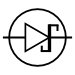There are two main differences between a schottky diode and a normal diode. One – a schottky diode does not have a discernible recovery time between conducting and not conducting a current. For example, a normal diode may take around a few hundred nanoseconds; whereas a schottky does not. This makes them useful in situations that involve very very high speed switching of current (for example, DC-DC converters such as Limor Fried’s mintyboost). Two – a schottky diode has a smaller forward voltage, a typical example (data sheet) is 0.55v.

Finally we come to rectifier diodes. Their main feature is the ability to handle large amounts of current, from 1 amp upwards; and higher breakdown voltages. For example the 1N4001 (data sheet) diode is 50V at 1 amp; the 1N5401 (data sheet) is 100V at 3 amps. The main purpose of these diodes is to protect against incorrect polarity from power supplies, and to convert AC to DC. For example, if you were designing a childrens’ toy that used a 9V battery, you would use reverse-bias a rectifier diode between 9V and GND in case the child forced the battery in the wrong way.

But how can rectifier diodes convert AC to DC power? Very easily – through the use of a bridge rectifier. A bridge rectifier is basically four rectifier diodes connected together, for example:When the AC power is between 0 and maximum wave, the positive DC rail is fed by the path: 1,2,3,4; the negative DC rail is 8,7,6,5. When the AC power is between 0 and minimum wave, the positive DC rail is fed by the path: 5,6,3,4; the negative DC rail is: 8,7,2,1.

Bridge rectifiers come in various shapes and sizes, for example DIP packaging for 1A 100V models: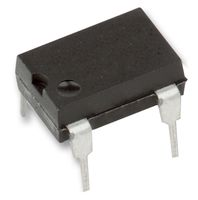right through to 300A 1600V models…

Last but not least is the light emitting diode (LED). An LED is a special kind of diode, when it is forward-biased and a current applied, it releases energy in the form of light instead of heat. Here is the common schematic symbol for an LED: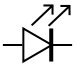When using an LED it is critical to ensure you have the correct voltage, otherwise your LED will overheat, burn your fingers when you touch it then eventually break. Always consult your data sheet. Calculating the correct voltage is quite simple. Using a bog-standard 5mm RED LED as an example (data sheet), you can use the following formula:

R = (Vs-Vled) / A

where:

• R = value of resistor to use in ohms
• Vs is your supply voltage in volts DS
• Vled is the forward voltage of the LED at the recommended current
• A is the recommended operation current of the LED

So for our example, we will use a 9V battery, and the LED from the data sheet above, Vled is 2V and A is 20 mA or 0.02 A

That gives us R = (9-2)/0.02 = 7/0.02 = 350 ohms.

Therefore, place a 350 ohm resistor between the positive of the battery and the anode of the LED. The most popular value of resistor to use would be a 390 ohm, 1/4 watt.

You can find LEDs in many different colours, and also units with two or more LEDs in the one housing, example red, green and blue. Some LEDs also create light in non-visible wavelengths, such as infra-red – these are used in remote-control applications and night-vision equipment. However if you are reading this, you would know by now where to find LEDs.

Well that wraps up my introduction to diodes. And if you made it this far – check out my new book “Arduino Workshop” from No Starch Press.

In the meanwhile have fun and keep checking into tronixstuff.com. Why not follow things on twitterGoogle+, subscribe  for email updates or RSS using the links on the right-hand column? And join our friendly Google Group – dedicated to the projects and related items on this website. Sign up – it’s free, helpful to each other –  and we can all learn something.

1. […] AC current is converted to DC current using a bridge rectifier (four diodes) the resulting DC current is not very smooth… that is the actual voltage changes between zero […]

2.Rodrigo says:

hi, what happens if i use a standard rectifier diode as a 1n4007 instead of a signal diode for a transistor protection?

•John Boxall says:

You need to make sure the 1n4007 can handle the powerof the electrical signal, and also the frequency if the switching speed is high. Surge current handling ability decreases as the frequency of current application increases.

3.marcos assis says:

Hi! Could you gently expand on the relay coil topic? I thinked the coil sourced more current, but it’s not, caus the signal diode handles low current. Is there any other text about realy circuits? If not, this would be a great topic for one tutorial! You can do many wonderful thing with realys (including solid state ones).
[]s

•John Boxall says:

An articles on relays? I will look into it.
john

4.girum says:

Great topic. if you are using a 125V dc source on a bridge to prevent a back feed. is it possible to have a ground on the source from the output side? in otherword,
I guess if you have a ground on a 125VDC source could it be caused by the short on the output side? I hope it makes sense.